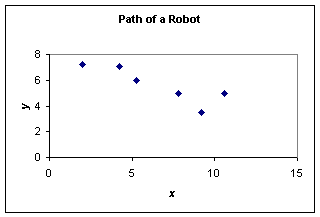# Quiz Chapter 05.05: Spline Method of Interpolation

 MULTIPLE CHOICE TEST BACKGROUND INTERPOLATION

1. The following $n$ data points, $\left( x_{1}, y_{1} \right), \, \left( x_{2}, y_{2} \right), \, ... \, , \, \left( x_{n}, y_{n} \right)$ are given. For conducting quadratic spline interpolation the $x$-data needs to be

2. In cubic spline interpolation,

3. The following incomplete $y$ vs. $x$ data is given

 $x$ $1$ $2$ $4$ $6$ $7$ $y$ $5$ $11$ ???? ???? $32$

The data is fit by quadratic spline interpolants given by

• $f (x) = ax - 1, \, 1 \leq x \leq 2$
• $f (x) = -2x^{2} + 14x - 9, \, 2 \leq x \leq 4$
• $f (x) = bx^{2} + cx + d, \, 4 \leq x \leq 6$
• $f (x) = 25x^{2} - 303x + 928, \, 6 \leq x \leq 7$

where $a, \, b, \, c,$ and $d$, are constants. The value of $c$ is most nearly

4. The following incomplete $y$ vs. $x$ data is given

 $x$ $1$ $2$ $4$ $6$ $7$ $y$ $5$ $11$ ???? ???? $32$

The data is fit by quadratic spline interpolants given by

• $f (x) = ax - 1, \, 1 \leq x \leq 2$
• $f (x) = -2x^{2} + 14x - 9, \, 2 \leq x \leq 4$
• $f (x) = bx^{2} + cx + d, \, 4 \leq x \leq 6$
• $f (x) = ex^{2} - fx + g, \, 6 \leq x \leq 7$

where $a, \, b, \, c, \, d, \, e, \, f,$ and $g$, are constants. The value of $\dfrac{df}{dx}$ at $x = 2.6$ is most nearly

5. The following incomplete $y$ vs. $x$ data is given

 $x$ $1$ $2$ $4$ $6$ $7$ $y$ $5$ $11$ ???? ???? $32$

The data is fit by quadratic spline interpolants given by

• $f (x) = ax - 1, \, 1 \leq x \leq 2$
• $f (x) = -2x^{2} + 14x - 9, \, 2 \leq x \leq 4$
• $f (x) = bx^{2} + cx + d, \, 4 \leq x \leq 6$
• $f (x) = 25x^{2} - 303x + 928, \, 6 \leq x \leq 7$

where $a, \, b, \, c,$ and $d$, are constants. What is the value of$\, \displaystyle\int_{1.5}^{3.5} f (x) \, dx$?

6. A robot needs to follow a path that passes through six data points as shown in the figure.To find the shortest path that is also smooth, you would recommend which of the following?